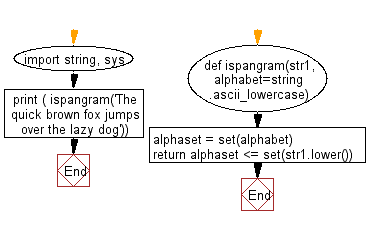﻿ Python Exercise: Check whether a string is a pangram or not - w3resource# Python Exercise: Check whether a string is a pangram or not

## Python Functions: Exercise-14 with Solution

Write a Python function to check whether a string is a pangram or not.

Note : Pangrams are words or sentences containing every letter of the alphabet at least once.
For example : "The quick brown fox jumps over the lazy dog"

Sample Solution:-

Python Code:

``````import string, sys
def ispangram(str1, alphabet=string.ascii_lowercase):
alphaset = set(alphabet)
return alphaset <= set(str1.lower())

print ( ispangram('The quick brown fox jumps over the lazy dog'))
```
```

Sample Output:

```True
```

Pictorial presentation:Flowchart:Python Code Editor:

Have another way to solve this solution? Contribute your code (and comments) through Disqus.

What is the difficulty level of this exercise?

Test your Programming skills with w3resource's quiz.

﻿

## Python: Tips of the Day

Decapitalizes the first letter of a string:

Example:

```def tips_decapitalize(s, upper_rest=False):
return s[:1].lower() + (s[1:].upper() if upper_rest else s[1:])
print(tips_decapitalize('PythonTips'))
print(tips_decapitalize('PythonTips', True))
```

Output:

```pythonTips
pYTHONTIPS
```